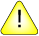This version of this document is no longer maintained. For the latest documentation, see http://www.qnx.com/developers/docs.

significand(), significandf()

Compute the "significant bits" of a floating-point number

Synopsis:

#include <math.h>

double significand ( double x );

float significandf ( float x );

Arguments:

x
A floating-point number.

Library:

libm

Use the -l m option to qcc to link against this library.

Description:

The significand() and significandf() functions are math functions that compute the "significant bits" of a floating-point number.

When encoding a floating-point number into binary notation, you remove the sign, and then shift the bits to the right or left until the shifted result is in the range [0.5, 1). The negative of the number of positions shifted is the exponent of the number, and the shifted result is the significand.

If x equals sig * 2n with 1 < sig < 2, then significand(x) returns sig for exercising the fraction-part(F) test vector. The function significand(x) isn't defined when x is one of:

• 0
• positive or negative infinity
• NAN.

Returns:

scalb ( x, (double) -ilogb (x) )

Since significand(x) = scalb(x, -ilogb(x)) where ilogb() returns the exponent part of x and scalb(x, n) returns a, such that x = a * 2 n, then:

When x is: scalbn(x, n) returns:
+/-infinity x
NAN NAN

Examples:

#include <stdio.h>
#include <errno.h>
#include <inttypes.h>
#include <math.h>
#include <fpstatus.h>

int main(int argc, char** argv)
{
double a, b, c, d;

a = 5;
b = ilogb(d);
printf("The exponent part of %f is %f \n", a, b);
c = significand(a);
printf("%f = %f * (2 ^ %f) \n", a, c, b);

return(0);
}

produces the output:

The exponent part of 5.000000 is -895.000000
5.000000 = 1.250000 * (2 ^ -895.000000)

Classification:

Unix

Safety:
Cancellation point No
Interrupt handler No
Signal handler No